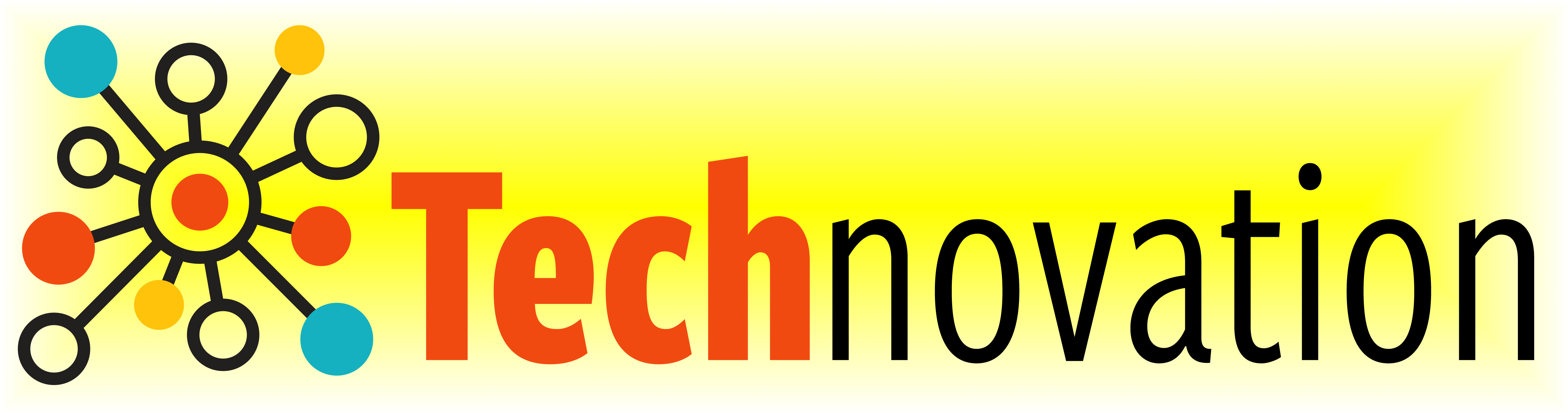# 7.3. Learning to Code: If Commands¶

## 7.3.1. Making code interactive¶

Remember user input last week? We can let the user decide what the turtle will draw by introducing an if command. Making a program interactive makes it a lot more fun!

For example, the code in the box below lets the user say if the `turtle` should draw a circle or not.

Run the code below twice. Enter `yes` at the prompt on one of the runs, and something else on the other. (After running it once, if you are not able to press the `Run` button, try refreshing the page.)

For the activity below, arrange the code blocks into a program that does the following, in order:

• defines a `draw_centered_circle` function as described by the docstring in the definition.

• defines a `draw_centered_square` function as described by the docstring in the definition.

• requests the user to enter either `square` or `circle`

• checks if the user entered `circle` and, if so, draws a circle

• checks if the user entered `square` and, if so, draws a square

• prints an error message if the user did not enter either `circle` or `square`

arrange the blocks

## 7.3.2. Making functions interactive¶

Recall the `draw_poly` function from the lesson on functions? Let’s revisit it to see how we can use the `if` command to make the `draw_poly` function both more interactive and more useful.

The definition of `draw_poly` from the lesson on functions appears below. Read over the function definition and the program that calls it. Then run the code.

Now suppose that we don’t want the polygons to be filled:Or suppose we want some filled, but not others:We could define another function that draws unfilled polygons and then call it instead of `draw_poly` when an unfilled polygon is needed. But that will create two functions that are almost identical, which is frowned on in coding. A better solution is to redefine function `draw_poly` to have a fourth parameter: a boolean value that indicates if the polygon should be filled or not. Say, we call this parameter `F`. We can then use if-commands so that `turtle.begin_fill()` and `turtle.end_fill()` commands are executed only if `F` equals `True`. This strategy will mean that the calls to `draw_poly` will need a fourth argument, either `True`, to draw a filled polygon, or `False`, to draw an unfilled polygon—e.g.,

`draw_poly( 10, 100, "purple", True)`.

We have started you out with a header for the new `draw_poly` definition below.

Add a body that uses the strategy described above. (Hint: Start with a copy of the old `draw_poly` body and replace the lines that call `turtle.begin_fill()` and `turtle.end_fill()` with if-commands.)

Once you have tested `draw_poly` and believe it is correct, use it to create the example diagrams above.

```if cond:
where cond stands for a boolean expression, or an expression that is either `True` or `False` and command1, command2, …, commandN stand for the commands that should be executed if cond equals `True`.